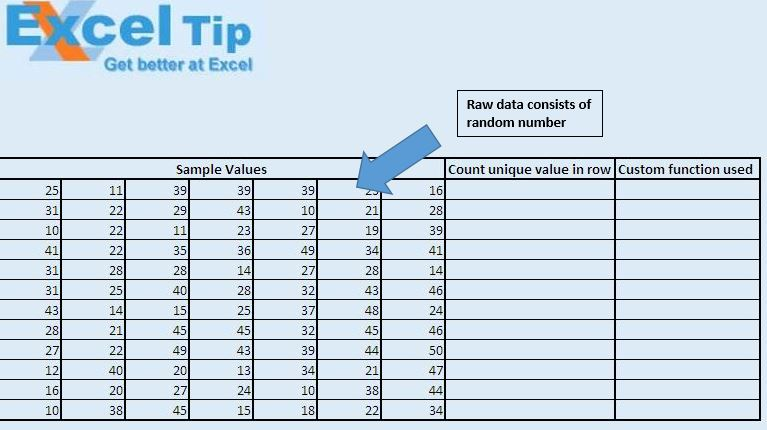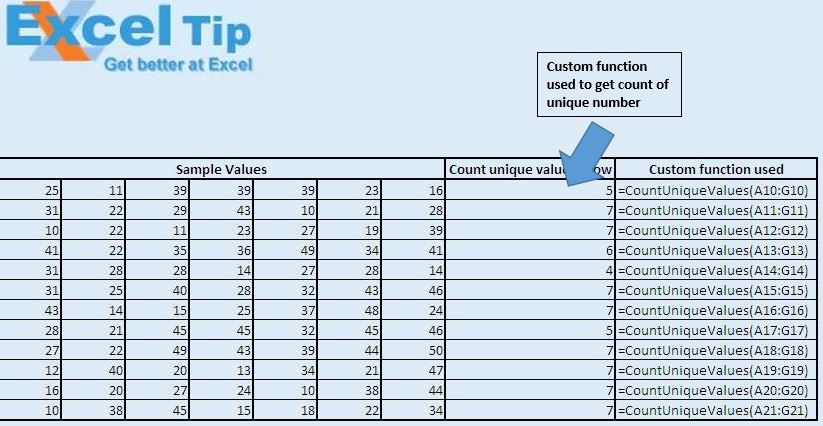# Count Unique Values Using VBA in Microsoft Excel 2010

In this article, we will create a custom function to count the unique values in the defined range.

Raw data for this example consists of random numbers in the rows. In this example, we want to count the number of unique numbers in each row.We have created “CountUniqueValues” custom function to find the count of unique numbers in the row. This function takes range as input and returns the unique count of numbers.Logic explanation

We have created custom function “CountUniqueValues” to get the count of unique numbers. In this custom function, we have created object of the collection object. This collection object is used to create the list of unique numbers. Once we have the list of unique numbers, we get the count of items in the collection.

Code explanation

Application.Volatile is used because it will recalculate every time a cell value is changed in the workbook.

On Error Resume Next is used to handle errors.

```Option Explicit

Function CountUniqueValues(InputRange As Range) As Integer

Dim CellValue As Variant, UniqueValues As New Collection

Application.Volatile

'For error Handling
On Error Resume Next

'Looping through all the cell in the defined range
For Each CellValue In InputRange
Next

'Returning the count of number of unique values
CountUniqueValues = UniqueValues.Count

End Function
```

We would love to hear from you, do let us know how we can improve our work and make it better for you. Write to us at info@exceltip.com

## Users are saying about us...

1."This is a good technique htat I have used a few times. I found it at JWalk, however I have also been caught out with it as it only works for ranges of one column or row in size - ie 1 x n or n x 1.

If anyone has a reliable UDF for n x m ranges, please let me know."

2."The function will work properly on ranges containing multiple rows and/or columns.
E.g. you can use it like this:
=CountUniqueValues(A1:D100) "

3."A better solution to this requirement is much easier! Just use the formula
=SUM(IF(FREQUENCY(A1:A100,A1:A100)>0,1)) in the cell that you want and it counts the unique values perfectly. Works across columns too. Its good for all kinds of cell data including dates and times. Just make sure the range is specified correctly (is the same) in both variables of the Frequency function."

4.I cannot get the Frequency function mentioned above to work. Could you give more details in using it and maybe provide an example using a list of names containing duplicates with the solution equaling a count of unique names in the list?

5.=SUM(IF(FREQUENCY(A1:A100,A1:A100)>0.1,1,0))

6.A little extention on Vikram's excellent solution. Thanks Vikram!!!!B90

7."I want to type in the cell (C4) and change that cell based upon what I type in that same cell (using value in C1)?
The following tip (vb) I got does not work - why??

Option Explicit

Private Sub Worksheet_Change(ByVal Target As Excel.Range)
If Target.Address = ""\$C\$4"" And Target.Value = ""Y"" Then
Target.Value = Range(""C1"").Value
End If
End Sub"

8.i cannot find the auto sum button as i dont know what it looks like.

9."I have a drop down list like this-
1. 248
2.238
3.ANT
4.86

These numbers or codes refer to individual product configuration. What I want to do is that when I select one of the codes, the worksheet I am working in gets populated with the product configuration corresponding the selected code."

10."1) Open any workbook (or a new one).

2) Click on Tools, Customise

3) Click on the Commands Tab

4) Select the Insert category

5) In the list of commands on the right, scroll down, and you will see the auto sum symbol with the narrative ""Auto Sum"" beside it.

It is eighth in my list using Excel 2000, may be different place for you. "

11."Have you looked at using LOOKUPs to achieve this?

Presumably the product code gets dropped into a cell on your worksheet, so you could then populate the rest of the cells using that as the reference. "

12."This is a good technique htat I have used a few times. I found it at JWalk, however I have also been caught out with it as it only works for ranges of one column or row in size - ie 1 x n or n x 1.

If anyone has a reliable UDF for n x m ranges, please let me know."

13.A little extention on Vikram's excellent solution. Thanks Vikram!!!!

14."This is a good technique htat I have used a few times. I found it at JWalk, however I have also been caught out with it as it only works for ranges of one column or row in size - ie 1 x n or n x 1.

If anyone has a reliable UDF for n x m ranges, please let me know."

15."The function will work properly on ranges containing multiple rows and/or columns.
E.g. you can use it like this:
=CountUniqueValues(A1:D100) "

16."A better solution to this requirement is much easier! Just use the formula
=SUM(IF(FREQUENCY(A1:A100,A1:A100)>0,1)) in the cell that you want and it counts the unique values perfectly. Works across columns too. Its good for all kinds of cell data including dates and times. Just make sure the range is specified correctly (is the same) in both variables of the Frequency function."

17.I cannot get the Frequency function mentioned above to work. Could you give more details in using it and maybe provide an example using a list of names containing duplicates with the solution equaling a count of unique names in the list?

18."SUM(IF(FREQUENCY(A1:A100,A1:A100)>0.1,1,0))
A little extention on Vikram's excellent solution. Thanks Vikram!!!!"

19.Hi all,
Looking at your function above and trying to combine it with a sumproduct but having some difficulty.
I need to count unique values in column D based on a value in column A (which can appear several times as well). Column A is File Name, Column D is Finding Number.
I have =SUMPRODUCT(Table_owssvr[File Names])*(CountUniqueValues(Table_owssvr[Finding Number]))

One other twist is that this workbook is actually an export from a SharePoint list which is then again read by some web parts to create some charts/metrics on a page on the SharePoint site. Hence a pivot table doesn't work - I can't write a formula to read the results of a pivot table.

Any suggestions is greatly appreciated.

20.Public Function Unique_Vals(r As Range) As Integer
'This function counts the number of unique members of a list
Dim uArray() As Variant
'starts the array
ReDim uArray(0)
'loops through each member of the range
For Each X In r
InList = False
'loops through all members of LotArray
If Not IsEmpty(X) Then
For Each n In uArray()
'compares x against all the members of LotArray
If (X = n) Then
InList = True
Exit For
End If
Next n
'If it's not in the list, adds it to it and increases upper bound of Lotarray
If InList = False Then
uArray(UBound(uArray)) = X
ReDim Preserve uArray(UBound(uArray) + 1)
End If
End If
Next
'gives count of lotarray
Unique_Vals = UBound(uArray)
End Function

21.Does anyone know the vba code to count the number of unique values in a range, but conditional on criteria in separate ranges (e.g. columns). So a function like:

CountUnique(range to count, criteria 1, criteria range 1, criteria 2, criteria range 2), etc.?

Terms and Conditions of use

The applications/code on this site are distributed as is and without warranties or liability. In no event shall the owner of the copyrights, or the authors of the applications/code be liable for any loss of profit, any problems or any damage resulting from the use or evaluation of the applications/code.